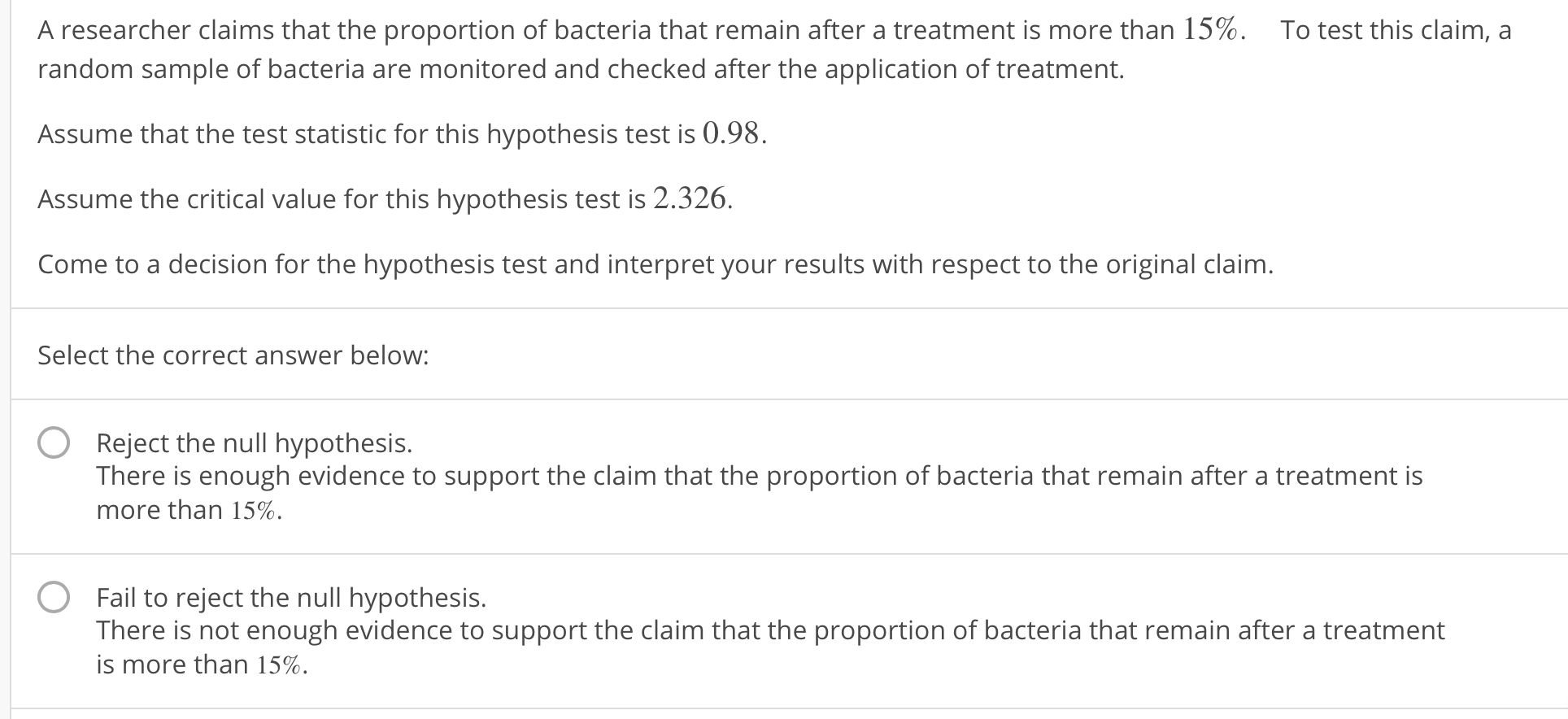# A researcher claims that the proportion of bacteria that remain after a treatment is more than 15%.To test this claim, arandom sample of bacteria are monitored and checked after the application of treatment.Assume that the test statistic for this hypothesis test is 0.98.Assume the critical value for this hypothesis test is 2.326.Come to a decision for the hypothesis test and interpret your results with respect to the original claim.Select the correct answer below:OReject the null hypothesis.There is enough evidence to support the claim that the proportion of bacteria that remain after a treatment ismore than 15%.OFail to reject the null hypothesis.There is not enough evidence to support the claim that the proportion of bacteria that remain after a treatmentis more than 15%.

Question
2 viewshelp_outlineImage TranscriptioncloseA researcher claims that the proportion of bacteria that remain after a treatment is more than 15%. To test this claim, a random sample of bacteria are monitored and checked after the application of treatment. Assume that the test statistic for this hypothesis test is 0.98. Assume the critical value for this hypothesis test is 2.326. Come to a decision for the hypothesis test and interpret your results with respect to the original claim. Select the correct answer below: O Reject the null hypothesis. There is enough evidence to support the claim that the proportion of bacteria that remain after a treatment is more than 15%. O Fail to reject the null hypothesis. There is not enough evidence to support the claim that the proportion of bacteria that remain after a treatment is more than 15%. fullscreen
check_circle

Step 1

Test hypothesis:

Denote the population proportion of bacteria that remains after a treatment as P.

The investigator is interested to test whether the population proportion of bacteria that remains after a treatment is greater than 0.15 or not.

The hypotheses are given below:

Null hypothesis:

H0 : P ≤ 0.15

That is, the population proportion of bacteria that remains after a treatment is equal to 0.15.

Alternative hypothesis:

H1 : P > 0.15 (Right tail test).

That is, the population proportion of bacteria that remains after a treatment is greater than 0.15.

Step 2

Given information:

The test statistic value of the hypothesis test is z = 0.98.

The critical value for the hypothesis test is zα = 2.326.

Step 3

Decision rule:

Denote z as test statistic value and zα as the critical value.

Decision rule based on critical approach:

If z > zα, then reject the null hypothesis H0.

If z &l...

### Want to see the full answer?

See Solution

#### Want to see this answer and more?

Solutions are written by subject experts who are available 24/7. Questions are typically answered within 1 hour.*

See Solution
*Response times may vary by subject and question.
Tagged in

### Other